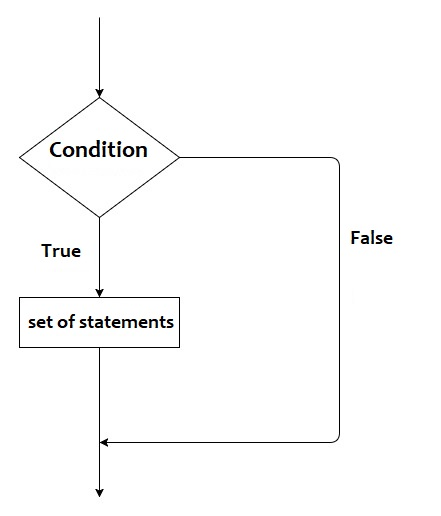# Golang if statement

Golang if statement: Learn about the simple if statement with syntax, flow chart, and examples.
Submitted by IncludeHelp, on December 10, 2021

The if statement is the simplest decision-making statement. It is used to check the given condition. If the given condition is true then the set of statements will be executed. If the condition is false then the set of statements will not be executed.

Syntax:

```if(condition){
// set of statements
}

or

if condition {
// set of statements
}
```

Flow chart:Example 1: Input the age of a person and check whether the person is eligible for voting or not.

In this program, we will use two if statements to check whether the person is eligible for voting or not.

```// Golang program to demonstrate the
// example of the simple if statement

package main

import "fmt"

func main() {
var age int

fmt.Print("Input the age: ")
fmt.Scanf("%d", &age)

if age >= 18 {
fmt.Println("Person is eligible for voting.")
}

if age < 18 {
fmt.Println("Person is not eligible for voting.")
}
}
```

Output:

```RUN 1:
Input the age: 32
Person is eligible for voting.

RUN 2:
Input the age: 12
Person is not eligible for voting.
```

Example 2: Input an integer value to check whether the given number is positive or negative.

In this program, we will use two if statements to check whether the given number is positive or negative.

```// Golang program to demonstrate the
// example of the simple if statement

package main

import "fmt"

func main() {
var num int

fmt.Print("Input an integer number: ")
fmt.Scanf("%d", &num)

if num >= 0 {
fmt.Println("Number is positive.")
}

if num < 0 {
fmt.Println("Number is negative.")
}
}
```

Output:

```RUN 1:
Input an integer number: 108
Number is positive.

RUN 2:
Input an integer number: -10
Number is negative.
```

Preparation

What's New

Top Interview Coding Problems/Challenges!